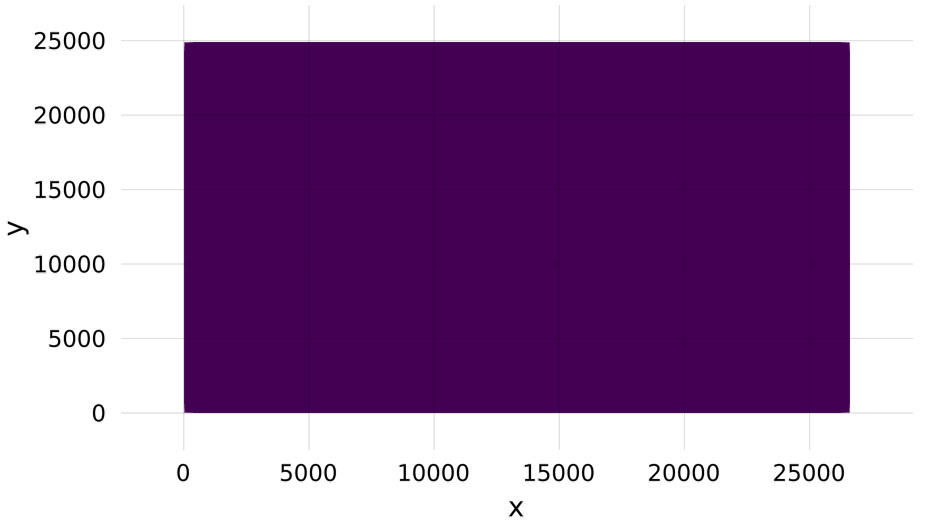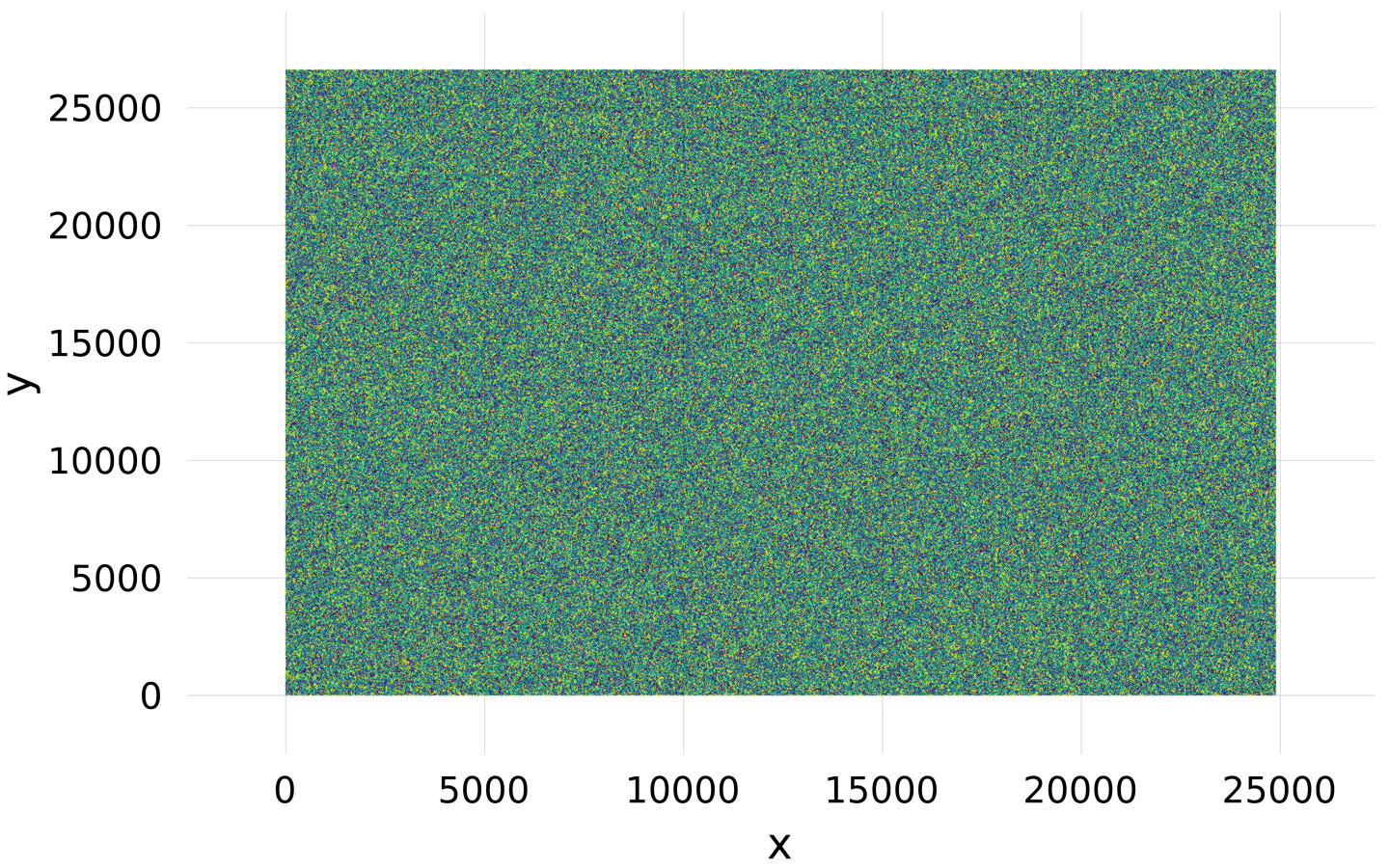# Frequently Asked Questions

## Installation Issues

We assume you are running Julia on the default system image without PackageCompiler.

### No `Scene` displayed or GLMakie fails to build

If `Makie` builds, but when plotting no window or plot is displayed, your backend may not have built correctly. By default, Makie will try to use GLMakie as a backend, but if it does not build correctly for whatever reason, then scenes will not be displayed. Ensure that your graphics card supports OpenGL; if it does not (old models, or relatively old integrated graphics cards), then you may want to consider CairoMakie.

## Plotting issues

### Dimensions too large

In general, plotting functions tend to plot whatever's given to them as a single texture. This can lead to GL errors, or OpenGL failing silently. To circumvent this, one can 'tile' the plots (i.e., assemble them piece-by-piece) to decrease the individual texture size.

#### 2d plots (heatmaps, images, etc.)

``heatmap(rand(Float32, 24900, 26620))``

may either fail with an error

``````Error showing value of type Scene:
ERROR: glTexImage 2D: width too large. Width: 24900
[...]``````

or fail silently:Tiling the plot, as shown below, yields a correct image.

``````sc = Scene()
data = rand(Float32, 24900, 26620)
heatmap!(sc, 1:size(data, 1)÷2, 1:size(data, 2)÷2, data[1:end÷2, 1:end÷2])
heatmap!(sc, (size(data, 1)÷2 + 1):size(data, 1), 1:size(data, 2)÷2, data[(end÷2 + 1):end, 1:end÷2])
heatmap!(sc, 1:size(data, 1)÷2, (size(data, 2)÷2 + 1):size(data, 2), data[1:end÷2, (end÷2 + 1):end])
heatmap!(sc, (size(data, 1)÷2 + 1):size(data, 1), (size(data, 2)÷2 + 1):size(data, 2),
data[(end÷2 + 1):end, (end÷2 + 1):end])``````#### 3d plots (volumes)

The approach here is similar to that for the 2d plots, except that here there is a helpful function that gives the maximum texture size. You can check the maximum texture size with:

``````using Makie, GLMakie, ModernGL
# simple plot to open a window (needs to be open for opengl)
display(scatter(rand(10)))
glGetIntegerv(GL_MAX_3D_TEXTURE_SIZE)``````

and then just split the volume:

``````vol = rand(506, 720, 1440)
ranges = (1:256, 1:256, 1:256)
scene = volume(ranges..., vol[ranges...])
for i in 1:3
global ranges
ranges = ntuple(3) do j
s = j == i ? last(ranges[j]) : 1
e = j == i ? size(vol, j) : last(ranges[j])
s:e
end
volume!(ranges..., vol[ranges...])
end
scene``````

## General issues

### My font doesn't work!

If `Makie` can't find your font, you can do two things:

1. Check that the name matches and that the font is in one of the directories in:

• `using FreeTypeAbstraction; FreeTypeAbstraction.valid_fontpaths`

2. You can add a custom font path via the environment variable:

• `ENV["FREETYPE_ABSTRACTION_FONT_PATH"] = "/path/to/your/fonts"`

3. Specify the path to the font; instead of `font = "Noto"`, you could write `joindir(homedir(), "Noto.ttf")` or something.

## Layout Issues

### Elements are squashed into the lower left corner

Block elements require a bounding box that they align themselves to. If you place such an element in a layout, the bounding box is controlled by that layout. If you forget to put an element in a layout, it will have its default bounding box of `BBox(0, 100, 0, 100)` which ends up being in the lower left corner. You can also choose to specify a bounding box manually if you need more control.

``````using CairoMakie

f = Figure()

ax1 = Axis(f, title = "Squashed")
ax2 = Axis(f[1, 1], title = "Placed in Layout")
ax3 = Axis(f, bbox = BBox(200, 600, 100, 500),
title = "Placed at BBox(200, 600, 100, 500)")

f``````### Columns or rows are shrunk to the size of Text or another element

Columns or rows that have size `Auto(true)` try to determine the width or height of all single-spanned elements that are placed in them, and if any elements report their size the row or column will shrink to the maximum reported size. This is so smaller elements with a known size take as little space as needed. But if there is other content in the row that should take more space, you can give the offending element the attribute `tellheight = false` or `tellwidth = false`. This way, its own size can be determined automatically, but it doesn't report it to the row or column of the layout. Alternatively, you can set the size of that row or column to `Auto(false)` (or any other value than `Auto(true)`).

``````using CairoMakie

f = Figure()

Axis(f[1, 1], title = "Shrunk")
Axis(f[2, 1], title = "Expanded")
Label(f[1, 2], "tellheight = true", tellheight = true)
Label(f[2, 2], "tellheight = false", tellheight = false)

f``````### The Figure content does not fit the Figure

`GridLayout`s work by fitting all their child content into the space that is available to them. Therefore, the `Figure` size determines how the layout is solved, but the layout does not influence the `Figure` size.

This works well when all content is adjustable in width and height, such as an `Axis` that can shrink or grow as needed. But it is also possible to constrain elements or rows/columns in width, height or aspect. And if too many elements have such constraints, it's not possible any longer to fit them all into the given `Figure` size, without leaving whitespace or clipping them at the borders.

If this is the case, you can use the function `resize_to_layout!`, which determines the actual size of the main `GridLayout` given its content, and resizes the `Figure` to fit.

Here is an example, where all `Axis` objects are given fixed widths and heights. There are not enough degrees of freedom for the layout algorithm to fit everything nicely into the `Figure`:

``````using CairoMakie

set_theme!(backgroundcolor = :gray90)

f = Figure(resolution = (800, 600))

for i in 1:3, j in 1:3
ax = Axis(f[i, j], title = "\$i, \$j", width = 100, height = 100)
i < 3 && hidexdecorations!(ax, grid = false)
j > 1 && hideydecorations!(ax, grid = false)
end

Colorbar(f[1:3, 4])

f``````As you can see, there's empty space on all four sides, because there are no flexible objects that could fill it.

But once we run `resize_to_layout!`, the `Figure` assumes the appropriate size for our axes:

``````resize_to_layout!(f)
f``````